Teaching support from the UK’s largest provider of in-school maths tuitionone to one lessonsschools supported

Deepen mathematical understanding

396 mastery-aligned teaching units designed to plug individual gaps and delivered by specialist tutors

See it in action

Hundreds of FREE online maths resources!

Daily activities, ready-to-go lesson slides, SATs revision packs, video CPD and more!Explore all resources
KS2 SATs
KS3 & KS4 Maths
Classroom Teaching
Maths Mastery
Maths Interventions
Home Learning

Deepen mathematical understanding

396 mastery-aligned teaching units designed to plug individual gaps and delivered by specialist tutors

See it in action

# The Ultimate Guide To The Bar Model: How To Teach It And Use It In KS1 And KS2

Teaching the bar model in maths and different bar modelling techniques in KS2 and KS1 is essential if you want pupils to do well in their reasoning and problem solving. Here’s your step by step guide to how to teach the bar model as part of maths mastery lessons from early years through KS1 SATs right up to KS2 SATs type questions.

First we will look at the four operations and a progression of bar model representations that can be applied across school in Key Stage 1 and Key Stage 2.

Then we will look at more complex bar model examples for KS2 SATs, including how to apply bar models to other concepts such as fraction and equations.

Finally, look out at the end of the article for some further bar model worksheets and free resources to get you started.

### What is a bar model in maths?

What is a bar model? In maths a bar model is a pictorial representation of a problem or concept where bars or boxes are used to represent the known and unknown quantities. Bar models are most often used to solve number problems with the four operations – addition and subtraction, multiplication and division.

In word problems, bar models help children decide which operations to use or visualise problems.

The bar model is central to maths mastery, the pictorial stage in the concrete pictorial abstract (CPA) approach to learning. Bar models will not, however, do the calculations for the pupil; they simply make it easier for pupils to work out which calculation must be done to solve the problem.

#### What is bar modelling in maths?

Bar modelling is the term used when you are teaching, learning or applying your bar models, and drawing out each bar to represent the known and unknown quantities. Encouraging a child to ‘bar model’ a problem can help them to understand conceptually what maths operation is required from the problem, and how each part combines to make the whole.

Here bar modelling shows us that if 2 rectangles out of 3 rectangles are green, then two thirds of 12 must be 8. An understanding of bar models is essential for teaching how to solve all sorts of word problems especially those using four operations (addition, subtraction, multiplication, division), fractions and algebra.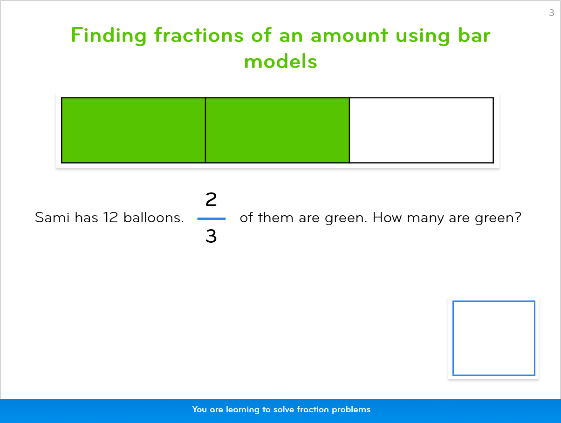An example bar model from Third Space Learning’s online intervention platform

#### Why use the bar model method in maths?

Bar modelling is much used in Singapore and Asian Maths textbooks and is an essential part of the mastery maths approach used by schools at all stages of the national curriculum.

By using the bar method to visualise problems, pupils are able to tackle any kind of number problem or complex word problem.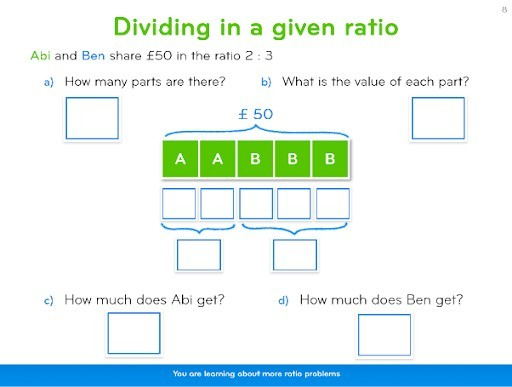A word problem from Third Space Learning’s online platform showing how to represent ratios with a bar model.

Because bar models only require pencils and paper, they are highly versatile and can come in very useful for tests, especially SATs Reasoning Papers.

However the use of bar models can begin much earlier, from showing number bonds to ten or partitioning numbers as part of your place value work.

Once a child is secure in their use of bar modelling for the four operations and can conceptualise its versatility, they can start to use it to visualise many other maths topics and problems, such as statistics and data handling.

#### The Singapore Bar Model Method

‘The Singapore Maths Model’ is another name for the bar model method. Despite this name however the Singapore bar model (like most of the maths mastery approach) is based heavily on the work of Bruner, Dienes and Bishop about the best way to help children learn: teaching for mastery.

Bar models act as a ‘bridge’ between the concrete, pictorial and abstract (CPA in maths); once children are secure with using pictorial versions of their concrete materials, they can progress to using bars as visual representations.

Bars are a more abstract way of representing amounts, making the transition to using wholly abstract numbers significantly less difficult.

#### The Part Whole Method

Also known as the ‘part part whole’ method or the comparison model, this kind of bar model uses rectangles to represent the known and unknown quantities as parts of a whole. This is an excellent method to help pupils represent the very common ‘missing number’ problems.

This can be done in two ways:

• As discrete parts to a whole – each unit in the problem has its own individual box, similar to using Numicon cubes.
• As continuous parts to a whole – units are grouped into one box for each amount in the problem e.g. in 26 + 52, 26 would have one long bar, not 26 smaller rectangles joined together.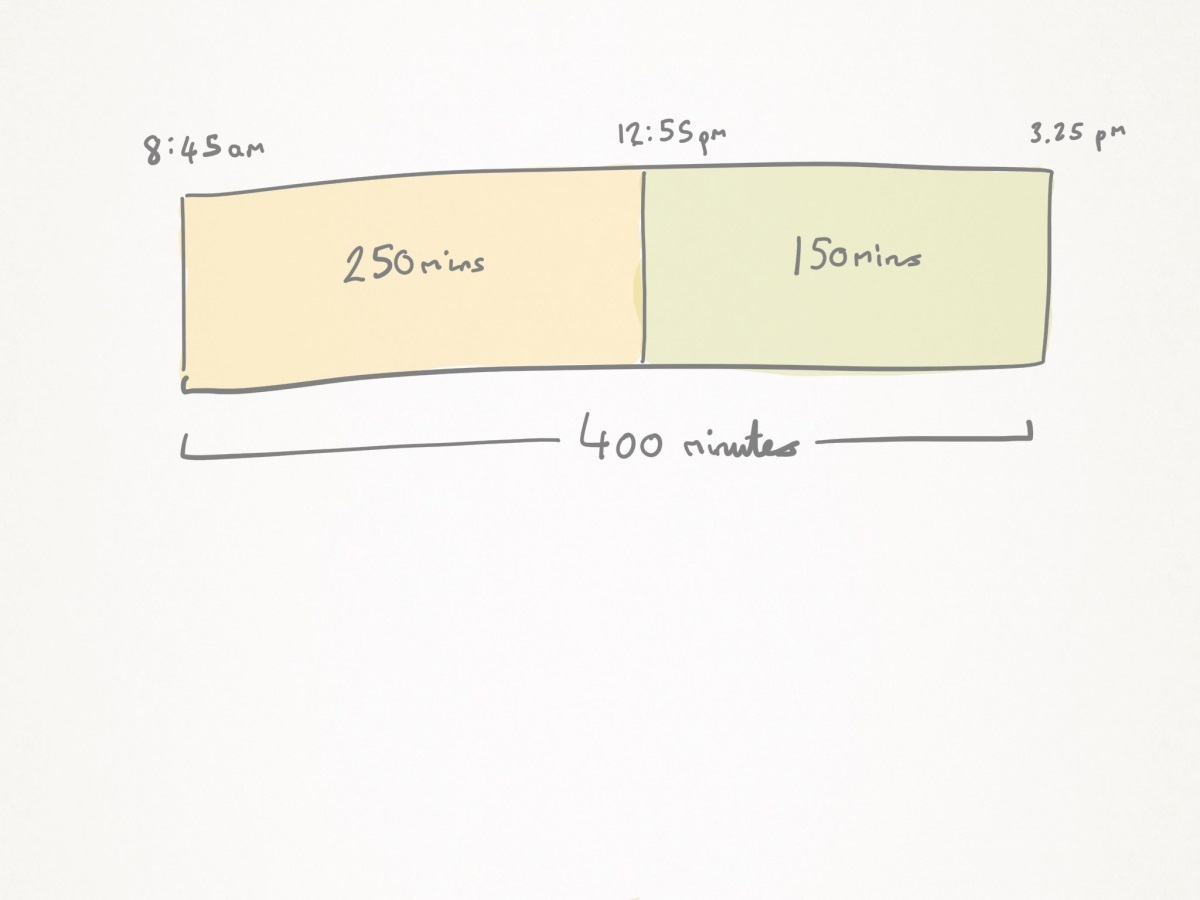An example of a continuous part-whole bar model from the Third Space Learning resource Let’s Practise Bar Modelling.

When using the part-whole method, proportionality is key; all the bars must be roughly proportional to each other e.g. 6 should be about twice the length of 3. Often you’ll find this is referred to as the

Part-whole models are generally used to visually represent the four operations, fractions, measure, algebra and ratio, but can be applied to many more topics (as long as they’re relevant!).

Read more: What Is The Part Whole Model?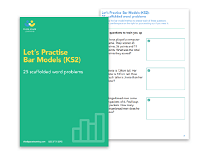[FREE] Let's Practise Bar Model Word Problems KS2

25 scaffolded bar model word problems on the four operations. Questions suitable for Year 3 to Year 6.Download Free

### How to draw a bar model

There are a few steps involved in drawing a bar model and using it to solve a problem:

1. Read the question carefully
2. Circle the important information
3. Determine the variables: who? what?
4. Make a plan for solving the problem: what operation needs to be used?
5. Draw the unit bars based on the information
6. Re-read the problem to make sure that the bar models match the information given
7. Complete the calculation using the determined operation.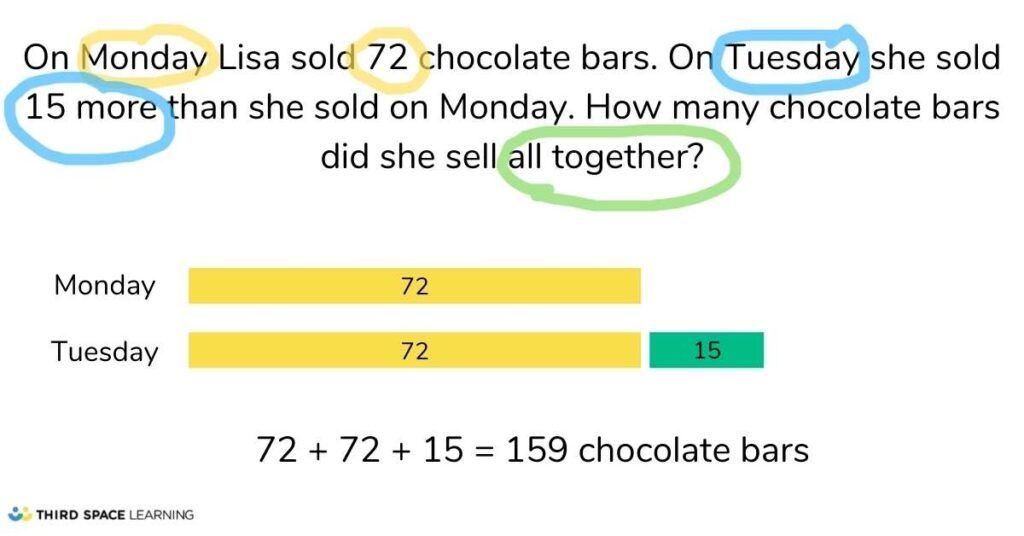Example question using steps of drawing a bar model

### Bar models KS1

#### Bar model addition KS1

Pupils in Reception and Year 1 will routinely come across calculations such as 4+3.

Often, these calculations will be presented as word problems: Aliya has 4 oranges. Alfie has 3 oranges. How many oranges are there altogether? With addition, subtraction and multiplication, to help children fully understand later stages of bar modelling, it is crucial they begin with concrete representations.

There are 2 models that can be used to represent addition:

Once they are used to the format and able to represent word problems with models in this way themselves (assigning ‘labels’ verbally), the next stage is to replace the ‘real’ objects with objects that represent what is being discussed (in this case, we replace the ‘real’ oranges with button counters):

The next stage is to move away from the concrete to the pictorial. As with all the stages, when pupils are ready for the next stage is a judgement call that is best decided upon within your school.

A general rule of thumb would be that towards the end of Year 1 or start of Year 2, pupils should be able to understand and represent simple addition (and subtraction) word problems pictorially and assign written labels in a bar model.

The penultimate stage is to represent each object as part of a bar, in preparation for the final stage:

The final stage stops the 1:1 representation. Each quantity is represented approximately as a rectangular bar:

As mentioned before, it is a judgement call for your school to make, but if you want pupils to use the bar model to support them in end of Key Stage 1 SATs tests, they are going to need to have had a fair amount of experience of this final stage.

#### Bar model subtraction KS1

The same concrete to pictorial stages can be applied to subtraction. However, whereas with addition it is really down to the pupil’s preference as to which of the 2 bar representations to use, with subtraction the teacher can nudge to pupils to one or other.

The reason? One represents a ‘part-part-whole’ model, the other a ‘find the difference’ model. Each will be more suited to different word problems and different pupils. Let’s examine those at the final stage of bar modelling:

##### Part-part-whole

Austin has 18 lego bricks. He used 15 pieces to build a small car. How many pieces does he have left?

Calculation: 18 – 15 =

##### Find the difference

Austin has 18 lego bricks. Lionel has 3 lego bricks. How many more lego bricks does Austin have than Lionel?

Calculation: 18 – 3 =

#### Bar model multiplication KS1

Bar model multiplication starts with the same ‘real’ and ‘representative counters’ stages as addition and subtraction. Then moves to its final stage, drawing rectangular bars to represent each group:

Each box contains 5 cookies. Lionel buys 4 boxes. How many cookies does Lionel have?

#### Bar model division KS1

Due to the complexity of division, it is recommended to remain grouping and sharing until the final stage of bar modelling is understood. Then word problems such as the 2 below can be introduced:

##### Sharing

Grace has 27 lollies. She wants to share them into 9 party bags for her friends. How many lollies will go into each party bag?

##### Grouping

Grace has 27 lollies for her party friends. She wants each friend to have 3 lollies. How many friends can she invite to her party?

#### Bar modelling for the KS1 SATs

Now that we have established a structure across school that allows for children to use bar models for KS1 SATs, we are now ready to teach pupils how to use the bar model for a deeper understanding of complex problems during Key Stage 2 and particularly in preparation for KS2 SATs.

The key question at any stage, at any age is what do we know? By training pupils to ask this when presented with word problems themselves, they quickly become independent at drawing bar models.

For example, in the problem: Egg boxes can hold 6 eggs. We need to fill 7 boxes. How many eggs will we need?

We know that there will be 7 egg boxes, so we know we can draw 7 rectangular bars. We know that each box holds 6 eggs, so we can write ‘6 eggs’ or ‘6’ in each of those 7 rectangular bar. We know we need to find the amount of eggs we have altogether. We can see we will need to use repeated addition or multiplication to solve the problem.

### Bar models KS2

#### Bar model for four operations word problems in KS2

Bar modelling can be used to solve Year 6 word problems. Let’s ramp up the difficulty a little. In a sample KS2 SATs, pupils are asked:

A bag of 5 lemons costs £1. A bag of 4 oranges costs £1.80. How much more does one orange cost than one lemon?

Pupils could represent this problem in the below bar model, simply by asking and answering ‘what do we know?’ This can

From here it should be straightforward for the pupils to ‘see’ or visualise their next step. Namely, dividing £1.80 by 4 and £1 by 5. Some pupils will not need the bar model to represent the next stage, but if they do, they would calculate and then allocate the cost onto the model:

Then those pupils that needed this stage, should be able to see that to answer the question, they need to calculate 45p – 20p. With the answer of 25p.

Download more bar modelling questions: Let’s Practise Bar Models Four Operations

#### Bar model for word problems with fractions in KS2

Here’s another example from the sample key stage 2 tests involving fractions and how it can be solved using a fraction bar model. On Saturday Lara read two fifths of her book. On Sunday, she read the other 90 pages to finish the book. How many pages are there in Lara’s book? If we create our bar model for what we know:

Pupils will then see that they can divide 90 by 3:

As fractions are ‘equal parts’ – a concept they should be familiar with from key stage 1 – they know that the other 2 fifths (Saturday’s reading) will be 30 pages each:

Then they can calculate 30 x 5 = 150

Download more bar modelling questions: Let’s Practise Bar Model Fractions

#### Equations with the bar model in KS2

There are lots of other areas bar models can assist pupil’s understanding such as ratio, percentages and equations. In this final example, we look at how an equation can be demystified using the comparison model:

2a + 7 = a + 11

Let’s draw what we know in a comparison model, as we know both sides of the equation will equal the same total:

The bars showing 7 and 11 could have been a lot smaller or larger as we don’t know their relative value to ‘a’ at this stage. However, it is crucial that the ‘a’ appearing first in both bars is understood to be equal (even if it is only approximately equal when drawn freehand in the bar). This allows the pupil to ‘see’ that to work out the second ‘a’ in the top bar, they can calculate 11-7.

So if that ‘a’ is 4, then both the other ‘a’s will also be 4. So each side of the equation will total 15. The below model shows all sections completed. This is not necessary for the pupils to do, the representation is merely useful until they can see the steps necessary to calculate whatever they are faced with:

#### Bar modelling for the KS2 SATs

At the SATs level most maths problems require multiple steps to solve, and incorporate numerous mathematical concepts e.g. money, fractions, four operations etc.

While bar modelling can be used to represent all these steps at the same time, pupils may find it easier to identify each step and draw bars separately, forming the answer gradually.

While there are some multi-step word problems that cannot be solved using this method, bar modelling does make a significant difference in pupil’s ability to work through the SATs.

Where next?

Now you’re persuaded (we hope!) that bar modelling in maths is going to revolutionise problem solving across your school from early years onwards, here are some other bar modelling resources to help you out.

### Free bar modelling resources for your primary school

1. FREE Ultimate Guide to Bar Modelling (including a bar modelling ppt) which will give a structure that can be put in place across the whole school to enable teachers to teach pupils the bar model consistently.
2. FREE KS2 Bar Model Worksheet with blank bar models to support children who need a bit more help on using bar models for word problems

### Premium teaching resources for your primary school

1. Lots of Bar Modelling Worksheets on our Maths Hub, including those that look at the Bar Model for Multiplication, Bar Model for Division, Bar Model for Subtraction etc
2. Bar Model Training through the Bar Model CPD videos on our Maths Hub specifically about teaching bar models – Year 1 through to Year 6.

What are you waiting for? Get a staff meeting booked, and encourage your staff to calculate difficult problems using bar models to support. They’ll be sold instantly and will be racing to go out and teach bar models to their own KS1 and KS2 pupils.

If you don’t believe me, use this problem to grab their attention:

With or without bar models? Which is easier? My guess is your staff will be hooked!

More on bar modelling and maths mastery techniques

If you’re looking for more maths resources that help develop maths problem solving techniques, all the White Rose Maths lesson slides contain a mix of fluency, reasoning and problem solving work as well as following a structured CPA approach; and on the Third Space Maths Hub you’ll find resources like Rapid Reasoning (daily word problems), and plenty of worked examples.

Do you have pupils who need extra support in maths?
Every week Third Space Learning’s maths specialist tutors support thousands of pupils across hundreds of schools with weekly online 1-to-1 lessons and maths interventions designed to plug gaps and boost progress.

Since 2013 we’ve helped over 125,000 primary and secondary school pupils become more confident, able mathematicians. Learn more or request a personalised quote for your school to speak to us about your school’s needs and how we can help.##### Pete Richardson
Walton Le Dale Primary School
Pete is a passionate convert to a mastery approach, blending the best of Singapore, Shanghai & home grown pedagogy. He blogs for Third Space on Mastery, & more!

Related Articles

x#### [FREE] Let's Practise Bar Model Word Problems KS2

25 scaffolded bar model word problems on the four operations. Questions suitable for Year 3 to Year 6.

#### [FREE] Let's Practise Bar Model Word Problems KS225 scaffolded bar model word problems on the four operations. Questions suitable for Year 3 to Year 6.